Browse by Topic
Related Topics

# Mathematica 7, Johannes Kepler and Transcendental Roots

Everyone who has been through high-school mathematics knows about polynomial equations. But what about equations involving other functions? Say equations like x == 1 - Sin[x].

These are transcendental equations, and they show up in a zillion different mathematical application areas. But they’re rarely talked about—perhaps because in some sense they’ve been an embarrassment: mathematics has had very little to say about them.

Polynomial equations and the algebraic numbers that represent their solutions have been one of the great success stories of pure mathematics. Over the past half millennium, a huge mathematical structure has been built on polynomial equations.

But almost nothing has been done with transcendental equations.

It’s not that they’re not important. In fact, what many people consider the very first computer—made of wood by Wilhelm Schickard in 1623—was built specifically to help in getting solutions to equations of the form x == 1 - e Sin[x].

Johannes Kepler was in the process of constructing his Rudolphine astronomical tables—and his killer technology for finding the longitude of a planet at a given time required solving what’s now called Kepler’s equation: essentially the transcendental equation x == 1 - e Sin[x].

With considerable effort, and probably computer support, Kepler made a table of solutions to this equation: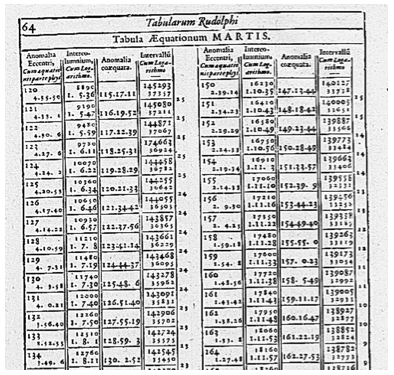Transcendental equations came up many times as mathematical physics developed—especially in boundary value and eigenvalue problems.

But unlike algebraic numbers, very little was figured out about the solutions to even very restricted classes of transcendental equations.

One of the innovations of Mathematica 3 was the introduction of Root[] objects that could symbolically represent any root of any polynomial equation—and could be manipulated symbolically like any other exact number.

About ten years ago we started to think about whether the concept of Root[] objects could be extended to transcendental equations.

At first it seemed daunting. There were theorems about undecidability everywhere (it’s easy to make an arbitrary Diophantine equation out of combinations of trigonometric functions). And there were almost no constructive general theorems.

There were signs of hope, however. Things like Weierstrass’s Factorization Theorem—which is a transcendental generalization of the Fundamental Theorem of Algebra—made it look as if there should be a systematic way to count and label roots for many transcendental equations.

A few years ago we started to have concrete ideas about how this might work. At first, they seemed quite impractical. But gradually we saw how to use all sorts of advanced symbolic and numerical computation features in Mathematica to make it practical.

And in Mathematica 7 we’ve brought everything together—to create for the first time a systematic treatment of a large class of transcendental roots.

Before Mathematica 7, we could only get exact solutions to equations that were reducible to polynomials (and inverse functions). But in Mathematica 7, with transcendental roots, the equations we could solve over the past 20 years is a set of measure zero relative to what we can solve today!

So how do we solve transcendental equations?

We start by looking at the class of holomorphic functions—essentially polynomials of infinite degree. A holomorphic function will often have infinitely many roots. But a key fact is that the roots are always countable—and, more importantly, there can only be finitely many roots in a given closed and bounded region.

Now we get to use some complex analysis. Given a region, we can tell how many roots are inside it either by directly computing the winding number around its boundary, or by using numerical integration and Cauchy’s theorem.

Once we have a count of the roots in a region we have a variety of methods for working out their rough specific locations. (Some methods are based on computational geometry and curve-curve intersections, some on complex interval arithmetic, and some on generalized eigenvalues derived from Cauchy’s theorem.) Given rough locations for roots, we then use Newton-like iterative methods to home in on the roots. And finally, we use Mathematica’s interval arithmetic to prove that there can only be one root inside each small region we’ve identified.

(There is some subtlety here. Proving zero equivalence is in general undecidable—and the way this shows up is that in pathological cases it can in principle require unboundedly much precision to distinguish multiple roots from closely spaced single roots.)

In a sense the fundamental problem of handling transcendental roots is finding a way to label them. And if we can get a region that is known to contain just one root, then we can label that root in Mathematica by giving an arbitrary-precision number whose value is the center of the region, and whose precision gives a bounding radius for the region.

Even before Mathematica 7, we had symbolic Root objects for representing roots of equation: Root[f,] had represented a root of the equation f[x]==0. But before Mathematica 7, f[x] had to be a polynomial.

Still, we had planned ahead. So in Mathematica 7 all we had to do was to say that in Root[f,], f[x] could be a transcendental function—and then invent a way of labeling different Root objects.

But having done this, we get a Root[] object that provides a precise symbolic description of any transcendental root—just like Sqrt or Pi^2 provide precise symbolic descriptions of numbers.

And because Mathematica is consistently symbolic, we can immediately use any of these symbolic descriptions of numbers wherever in the system we need a number—whether in equations or inequalities, or in linear algebra, or in the argument to a function, or anywhere.

In a sense what we’re doing with transcendental roots is to generalize Mathematica the same way that mathematics itself has been generalized.

First one has integers. Then one introduces rational numbers to solve linear equations. Then algebraic numbers to solve polynomial equations.

What Mathematica 7 now does is to introduce another class of “constructive numbers”—transcendental roots that solve transcendental equations. And then it gives us way to work with these transcendental roots.

Here’s an example. First, we use Reduce to go from an equation to a symbolic transcendental root.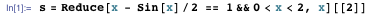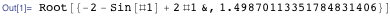We can evaluate the root to any precision.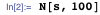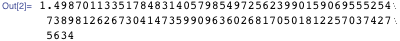Or we can just use it symbolically, and get exact results out.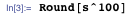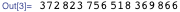Mathematica 7 has all sorts of ways of dealing with transcendental equations.

It has a whole repertoire of substitutions and transformations for taking equations that are presented in a transcendental way and reducing them to compositions of polynomial equations and functions with definite inverses.

Usually it does best when there is a finite range for the variables in a transcendental equation. But it can handle infinite ranges when there is periodicity in the functions that are involved.

And there are whole other classes of transcendental equations that Mathematica 7 handles by quite different methods. For example, it resolves nested exp-log equations over the reals by constructing Hardy fields and doing symbolic analysis of expression structures.

We’ve managed to get a surprisingly long way with transcendental equations in Mathematica 7. But of course, it’s not the end of story.

In Mathematica 7, we deal with holomorphic functions. We haven’t figured out how to deal with branch cuts, or accumulation points. Or multivariate functions. Or how to do RootReduce[] for combinations of transcendental roots.

But it’s very satisfying to see that some pure mathematics we’ve done has immediately introduced a whole new class of equations that can be handled symbolically—and has opened up all sorts of new application areas.

It’s also nice to realize that—after 385 years—Kepler’s killer technology for constructing astronomical tables can be reduced to just a single line of Mathematica 7 input.